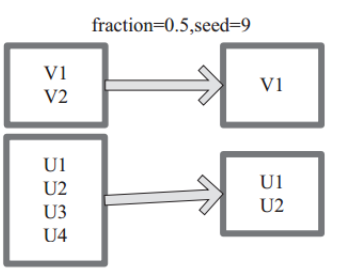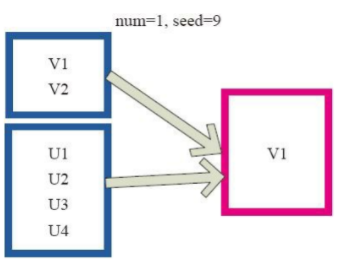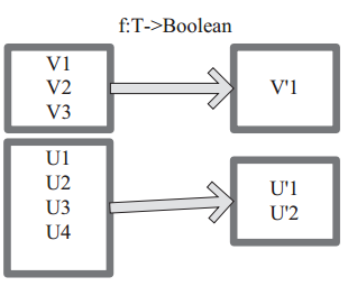cau red 所有 cep 調用 ext log 源碼 rsa

遇到的問題

> - 有序取 TopN
> - 無序取 N

• sample函數
• sample(boolean, fraction,seed) : 按比例抽取
• 返回一個新的RDD

withReplacement：元素可以多次抽樣(在抽樣時替換)
• withReplacement=true，表示有放回的抽樣
• withReplacement=false，表示無放回的抽樣

fraction：期望樣本的大小作為RDD大小的一部分， 當withReplacement=false時：選擇每個元素的概率;分數一定是[0,1] ； 當withReplacement=true時：選擇每個元素的期望次數; 分數必須大於等於0
seed：隨機數生成器的種子• takeSample函數
• takeSample(boolean, sampleNum,seed) : 按固定數量抽取
• 返回一個Array[T]； 該方法僅在預期結果數組很小的情況下使用，因為所有數據都被加載到driver的內存中
• takeSample函數先是計算fraction，也就是采樣比例，然後調用sample函數進行采樣，並對采樣後的數據進行collect()，最後調用take函數返回num個元素

withReplacement：元素可以多次抽樣(在抽樣時替換)

• withReplacement=true，表示有放回的抽樣
• withReplacement=false，表示無放回的抽樣

num：返回的樣本的大小
seed：隨機數生成器的種子• filter函數
• 函數功能是對元素進行過濾，對每個元素應用 f 函 數，返回值為 true 的元素在RDD中保留，返回值為 false 的元素將被過濾掉。 內 部 實 現 相 當 於 生 成 FilteredRDD(this，sc.clean(f))
• 若是單列無法過濾，可以手動設置過濾位
• 有點遺憾的是無法準確的取固定量的數

圖中每個方框代表一個 RDD 分區， T 可以是任意的類型。通過用戶自定義的過濾函數 f，對每個數據項操作，將滿足條件、返回結果為 true 的數據項保留。例如，過濾掉 V2 和 V3 保留了 V1，為區分命名為 V‘1。圖 filter 算子對 RDD 轉換

def takeSample(
withReplacement: Boolean,
num: Int,
seed: Long = Utils.random.nextLong): Array[T] =
{
val numStDev = 10.0
if (num < 0) {
throw new IllegalArgumentException("Negative number of elements requested")
} else if (num == 0) {
return new Array[T](0)
}
val initialCount = this.count()
if (initialCount == 0) {
return new Array[T](0)
}
val maxSampleSize = Int.MaxValue - (numStDev * math.sqrt(Int.MaxValue)).toInt
if (num > maxSampleSize) {
throw new IllegalArgumentException("Cannot support a sample size > Int.MaxValue - "         + s"\$numStDev * math.sqrt(Int.MaxValue)")
}
val rand = new Random(seed)
if (!withReplacement && num >= initialCount) {
return Utils.randomizeInPlace(this.collect(), rand)
}
val fraction = SamplingUtils.computeFractionForSampleSize(num, initialCount,        withReplacement)
var samples = this.sample(withReplacement, fraction, rand.nextInt()).collect()
// If the first sample didn‘t turn out large enough, keep trying to take samples;
// this shouldn‘t happen often because we use a big multiplier for the initial size
var numIters = 0
while (samples.length < num) {
logWarning(s"Needed to re-sample due to insufficient sample size. Repeat #\$numIters")
samples = this.sample(withReplacement, fraction, rand.nextInt()).collect()
numIters += 1
}
Utils.randomizeInPlace(samples, rand).take(num)
}